# How do I calculate the time difference between many values?

Member Posts: 2Learner I
edited June 2021 in Help
Hello everybody,
I want to calculate the production time of a machine per order (idOrder). There are a total of 53 orders.
In this example, I have to subtract the time of line number 408 from 399, since the machine was active at that time.

Can you tell me how I calculate the production time?

Best regards and thank you very much
Max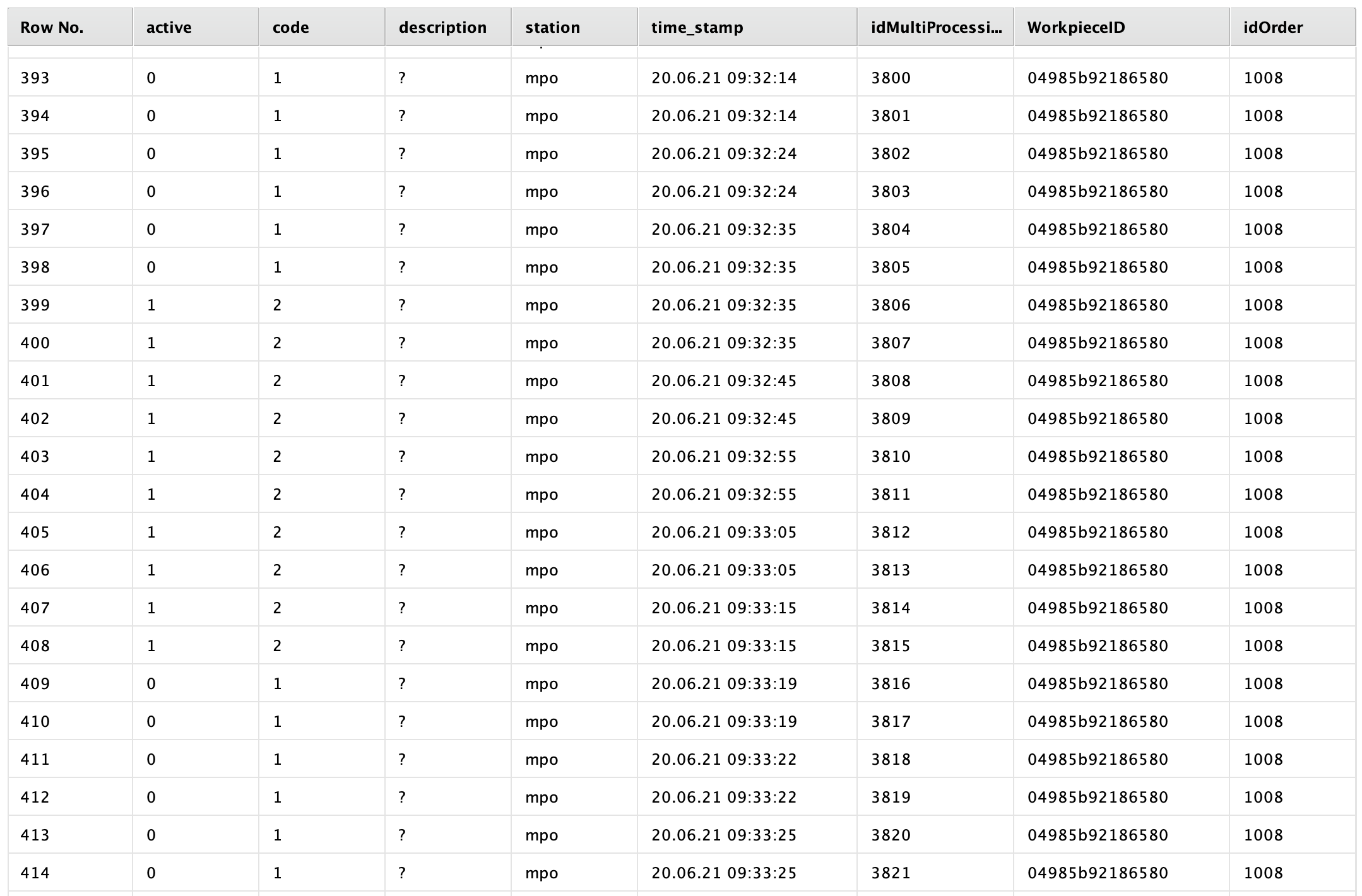Tagged:

• Moderator, RapidMiner Certified Analyst, Member, University Professor Posts: 568Unicorn
Solution Accepted
Hi, @MaxPr

What is the criteria used to know what are the values to substract? Is it the code?

I did the following with some sample data:

The data: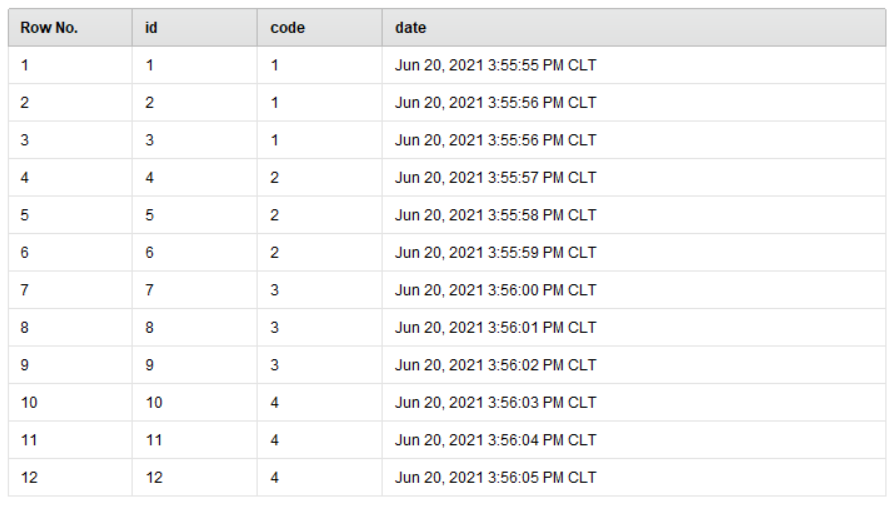I got that data, and applied two aggregations: the min(date), grouped by the code, and the max(date), again grouped by the code. Here is a screenshot.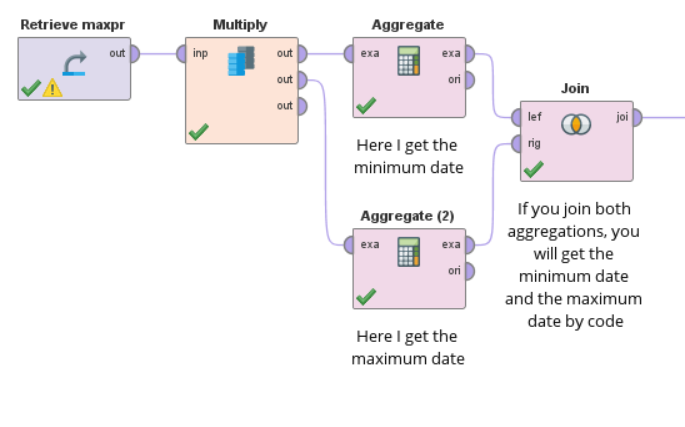When I joined these values, I got the following: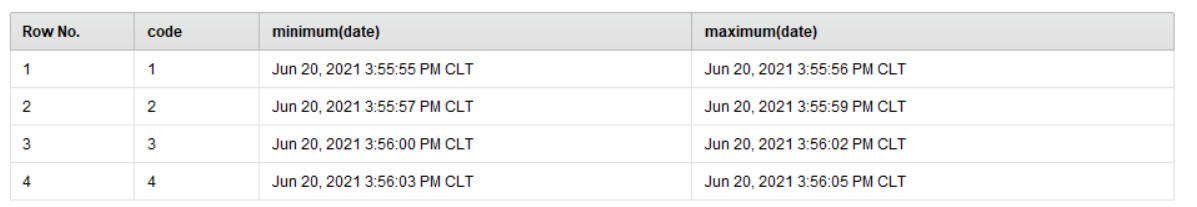And then I added another operator named "Generate Attributes" with the following configuration: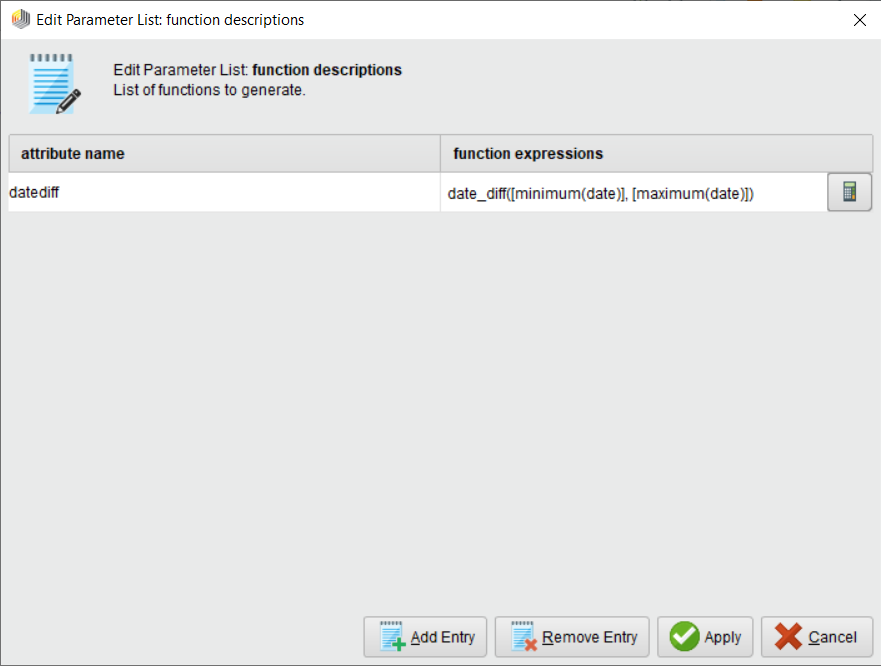The function expression to substract one date from another and get the number of seconds between the minimum date and the maximum one is the following:

floor(date_diff([minimum(date)], [maximum(date)])/1000)

(I got the screenshot before correcting the formula, so, sorry).

You can finally clean up and do whatever you want with that info.

Hope this helps. Please find attached the process and data too, if you wish to try it.

• Member Posts: 2Learner I
Solution Accepted
Hi @rfuentealba,
thanks for your answer. Your solution helped me a lot.